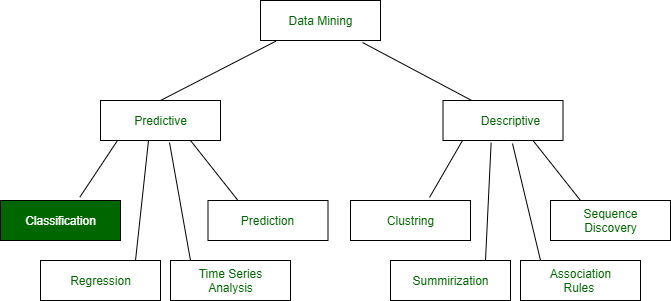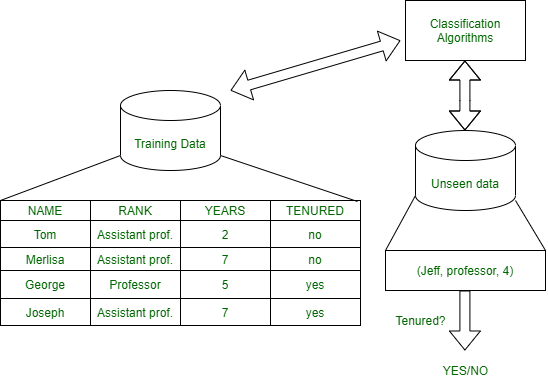GFG App
Open AppBrowser
Continue

# Basic Concept of Classification (Data Mining)

Data Mining: Data mining in general terms means mining or digging deep into data that is in different forms to gain patterns, and to gain knowledge on that pattern. In the process of data mining, large data sets are first sorted, then patterns are identified and relationships are established to perform data analysis and solve problems.

Classification is a task in data mining that involves assigning a class label to each instance in a dataset based on its features. The goal of classification is to build a model that accurately predicts the class labels of new instances based on their features.

There are two main types of classification: binary classification and multi-class classification. Binary classification involves classifying instances into two classes, such as “spam” or “not spam”, while multi-class classification involves classifying instances into more than two classes.

### The process of building a classification model typically involves the following steps:

Data Collection:
The first step in building a classification model is data collection. In this step, the data relevant to the problem at hand is collected. The data should be representative of the problem and should contain all the necessary attributes and labels needed for classification. The data can be collected from various sources, such as surveys, questionnaires, websites, and databases.

Data Preprocessing:
The second step in building a classification model is data preprocessing. The collected data needs to be preprocessed to ensure its quality. This involves handling missing values, dealing with outliers, and transforming the data into a format suitable for analysis. Data preprocessing also involves converting the data into numerical form, as most classification algorithms require numerical input.

Handling Missing Values: Missing values in the dataset can be handled by replacing them with the mean, median, or mode of the corresponding feature or by removing the entire record.

Dealing with Outliers: Outliers in the dataset can be detected using various statistical techniques such as z-score analysis, boxplots, and scatterplots. Outliers can be removed from the dataset or replaced with the mean, median, or mode of the corresponding feature.

Data Transformation: Data transformation involves scaling or normalizing the data to bring it into a common scale. This is done to ensure that all features have the same level of importance in the analysis.

Feature Selection:
The third step in building a classification model is feature selection. Feature selection involves identifying the most relevant attributes in the dataset for classification. This can be done using various techniques, such as correlation analysis, information gain, and principal component analysis.
Correlation Analysis: Correlation analysis involves identifying the correlation between the features in the dataset. Features that are highly correlated with each other can be removed as they do not provide additional information for classification.

Information Gain: Information gain is a measure of the amount of information that a feature provides for classification. Features with high information gain are selected for classification.

Principal Component Analysis:

Principal Component Analysis (PCA) is a technique used to reduce the dimensionality of the dataset. PCA identifies the most important features in the dataset and removes the redundant ones.

Model Selection:
The fourth step in building a classification model is model selection. Model selection involves selecting the appropriate classification algorithm for the problem at hand. There are several algorithms available, such as decision trees, support vector machines, and neural networks.
Decision Trees: Decision trees are a simple yet powerful classification algorithm. They divide the dataset into smaller subsets based on the values of the features and construct a tree-like model that can be used for classification.

Support Vector Machines: Support Vector Machines (SVMs) are a popular classification algorithm used for both linear and nonlinear classification problems. SVMs are based on the concept of maximum margin, which involves finding the hyperplane that maximizes the distance between the two classes.

Neural Networks:

Neural Networks are a powerful classification algorithm that can learn complex patterns in the data. They are inspired by the structure of the human brain and consist of multiple layers of interconnected nodes.

Model Training:
The fifth step in building a classification model is model training. Model training involves using the selected classification algorithm to learn the patterns in the data. The data is divided into a training set and a validation set. The model is trained using the training set, and its performance is evaluated on the validation set.

Model Evaluation:
The sixth step in building a classification model is model evaluation. Model evaluation involves assessing the performance of the trained model on a test set. This is done to ensure that the model generalizes well

Classification is a widely used technique in data mining and is applied in a variety of domains, such as email filtering, sentiment analysis, and medical diagnosis.Classification: It is a data analysis task, i.e. the process of finding a model that describes and distinguishes data classes and concepts. Classification is the problem of identifying to which of a set of categories (subpopulations), a new observation belongs to, on the basis of a training set of data containing observations and whose categories membership is known.

Example: Before starting any project, we need to check its feasibility. In this case, a classifier is required to predict class labels such as ‘Safe’ and ‘Risky’ for adopting the Project and to further approve it. It is a two-step process such as:

1. Learning Step (Training Phase): Construction of Classification Model
Different Algorithms are used to build a classifier by making the model learn using the training set available. The model has to be trained for the prediction of accurate results.
2. Classification Step: Model used to predict class labels and testing the constructed model on test data and hence estimate the accuracy of the classification rules.Test data are used to estimate the accuracy of the classification rule

Training and Testing:

Suppose there is a person who is sitting under a fan and the fan starts falling on him, he should get aside in order not to get hurt. So, this is his training part to move away. While Testing if the person sees any heavy object coming towards him or falling on him and moves aside then the system is tested positively and if the person does not move aside then the system is negatively tested.
The same is the case with the data, it should be trained in order to get the accurate and best results.

There are certain data types associated with data mining that actually tells us the format of the file (whether it is in text format or in numerical format).

Attributes – Represents different features of an object. Different types of attributes are:

1. Binary: Possesses only two values i.e. True or False
Example: Suppose there is a survey evaluating some products. We need to check whether it’s useful or not. So, the Customer has to answer it in Yes or No.
Product usefulness: Yes / No
• Symmetric: Both values are equally important in all aspects
• Asymmetric: When both the values may not be important.
2. Nominal: When more than two outcomes are possible. It is in Alphabet form rather than being in Integer form.
Example: One needs to choose some material but of different colors. So, the color might be Yellow, Green, Black, Red.
Different Colors: Red, Green, Black, Yellow
• Ordinal: Values that must have some meaningful order.
Example: Suppose there are grade sheets of few students which might contain different grades as per their performance such as A, B, C, D
• Continuous: May have an infinite number of values, it is in float type
Example: Measuring the weight of few Students in a sequence or orderly manner i.e. 50, 51, 52, 53
Weight: 50, 51, 52, 53
• Discrete: Finite number of values.
Example: Marks of a Student in a few subjects: 65, 70, 75, 80, 90
Marks: 65, 70, 75, 80, 90

Syntax:

• Mathematical Notation: Classification is based on building a function taking input feature vector “X” and predicting its outcome “Y” (Qualitative response taking values in set C)
• Here Classifier (or model) is used which is a Supervised function, can be designed manually based on the expert’s knowledge. It has been constructed to predict class labels (Example: Label – “Yes” or “No” for the approval of some event).

Classifiers can be categorized into two major types:

1. Discriminative: It is a very basic classifier and determines just one class for each row of data. It tries to model just by depending on the observed data, depends heavily on the quality of data rather than on distributions.
Example: Logistic Regression
2. Generative: It models the distribution of individual classes and tries to learn the model that generates the data behind the scenes by estimating assumptions and distributions of the model. Used to predict the unseen data.
Example: Naive Bayes Classifier
Detecting Spam emails by looking at the previous data. Suppose 100 emails and that too divided in 1:4 i.e. Class A: 25%(Spam emails) and Class B: 75%(Non-Spam emails). Now if a user wants to check that if an email contains the word cheap, then that may be termed as Spam.
It seems to be that in Class A(i.e. in 25% of data), 20 out of 25 emails are spam and rest not.
And in Class B(i.e. in 75% of data), 70 out of 75 emails are not spam and rest are spam.
So, if the email contains the word cheap, what is the probability of it being spam ?? (= 80%)

Classifiers Of Machine Learning:

1. Decision Trees
2. Bayesian Classifiers
3. Neural Networks
4. K-Nearest Neighbour
5. Support Vector Machines
6. Linear Regression
7. Logistic Regression

Associated Tools and Languages: Used to mine/ extract useful information from raw data.

• Main Languages used: R, SAS, Python, SQL
• Major Tools used: RapidMiner, Orange, KNIME, Spark, Weka
• Libraries used: Jupyter, NumPy, Matplotlib, Pandas, ScikitLearn, NLTK, TensorFlow, Seaborn, Basemap, etc.

RealLife Examples :

It is a modeling technique that has been associated with frequent transactions of buying some combination of items.
Example: Amazon and many other Retailers use this technique. While viewing some products, certain suggestions for the commodities are shown that some people have bought in the past.
• Weather Forecasting:
Changing Patterns in weather conditions needs to be observed based on parameters such as temperature, humidity, wind direction. This keen observation also requires the use of previous records in order to predict it accurately.

• Mining Based Methods are cost-effective and efficient
• Helps in identifying criminal suspects
• Helps in predicting the risk of diseases
• Helps Banks and Financial Institutions to identify defaulters so that they may approve Cards, Loan, etc.

Privacy: When the data is either are chances that a company may give some information about their customers to other vendors or use this information for their profit.
Accuracy Problem: Selection of Accurate model must be there in order to get the best accuracy and result.

APPLICATIONS:

• Marketing and Retailing
• Manufacturing
• Telecommunication Industry
• Intrusion Detection
• Education System
• Fraud Detection

GIST OF DATA MINING :

1. Choosing the correct classification method, like decision trees, Bayesian networks, or neural networks.
2. Need a sample of data, where all class values are known. Then the data will be divided into two parts, a training set, and a test set.

Now, the training set is given to a learning algorithm, which derives a classifier. Then the classifier is tested with the test set, where all class values are hidden.
If the classifier classifies most cases in the test set correctly, it can be assumed that it works accurately also on the future data else it may be the wrong model chosen.

My Personal Notes arrow_drop_up